# Summer School on Hashing 14 Locality Sensitive Hashing

• Slides: 31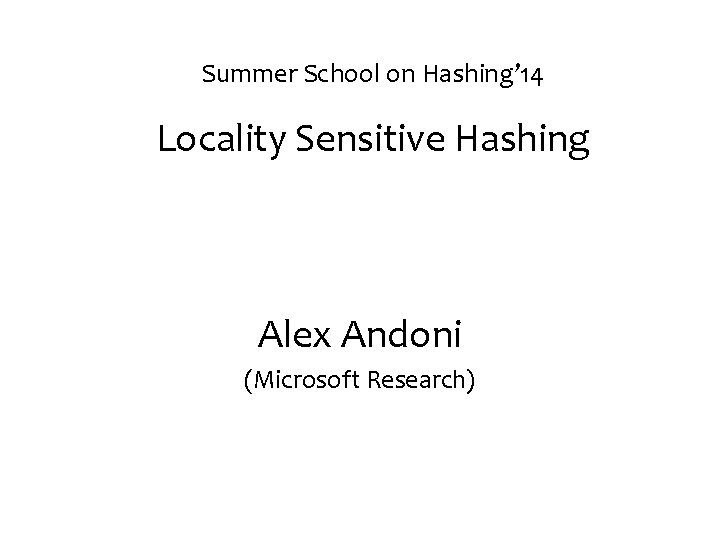Summer School on Hashing’ 14 Locality Sensitive Hashing Alex Andoni (Microsoft Research)Nearest Neighbor Search (NNS) •Approximate NNS e t a im x o r • -a pp c r cr q p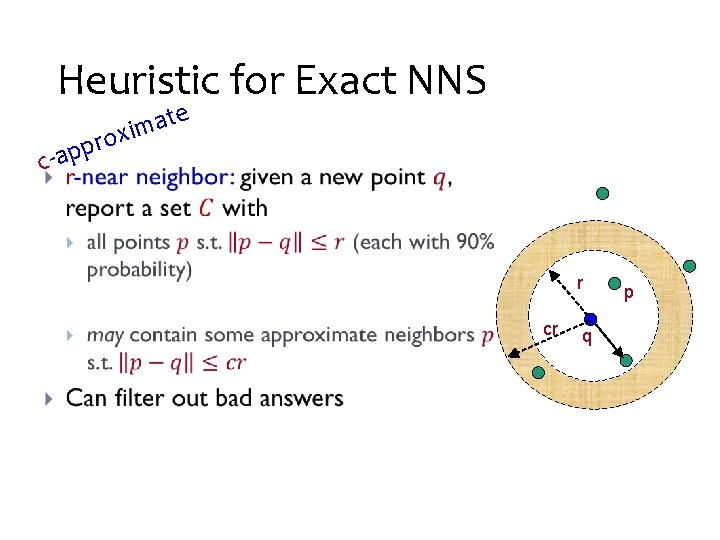Heuristic for Exact NNS e t a im x o r • -a pp c r cr q p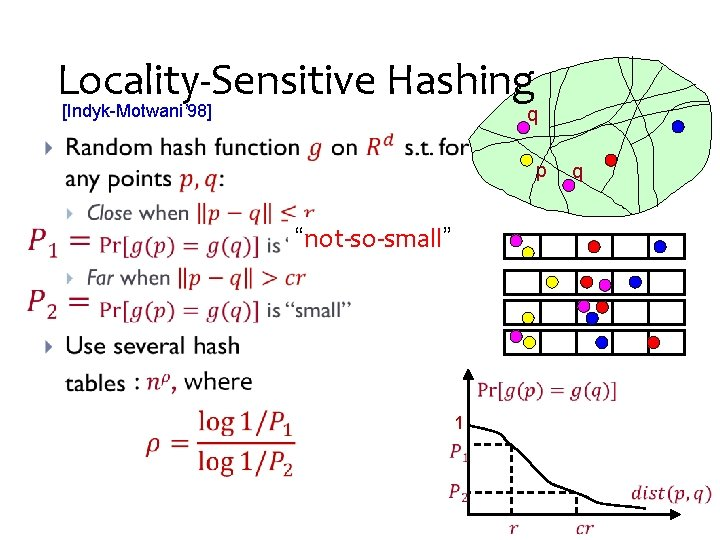Locality-Sensitive Hashing [Indyk-Motwani’ 98] q • p q “not-so-small” 1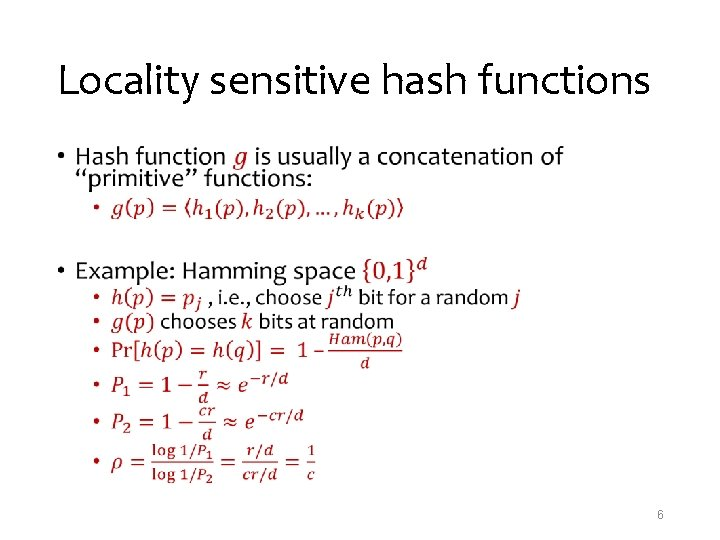Locality sensitive hash functions • 6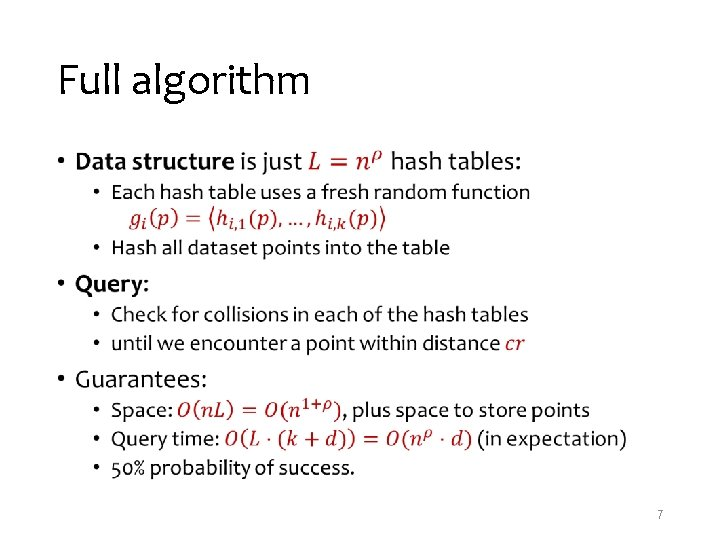Full algorithm • 7Analysis of LSH Scheme • collision probability distance 8Analysis: Correctness • 9Analysis: Runtime • 10NNS for Euclidean space [Datar-Immorlica-Indyk-Mirrokni’ 04] • 11Optimal* LSH [A-Indyk’ 06] • Regular grid → grid of balls p • p can hit empty space, so take more such grids until p is in a ball • Need (too) many grids of balls • Start by projecting in dimension t • Analysis gives • Choice of reduced dimension t? 2 D • Tradeoff between • # hash tables, n , and • Time to hash, t. O(t) • Total query time: dn 1/c 2+o(1) p Rtp Proof idea • Claim: , i. e. , • P(r)=probability of collision when ||p-q||=r • Intuitive proof: Projection approx preserves distances [JL] P(r) = intersection / union P(r)≈random point u beyond the dashed line Fact (high dimensions): the x-coordinate of u has a nearly Gaussian distribution → P(r) exp(-A·r 2) • • qq r p P(r) u x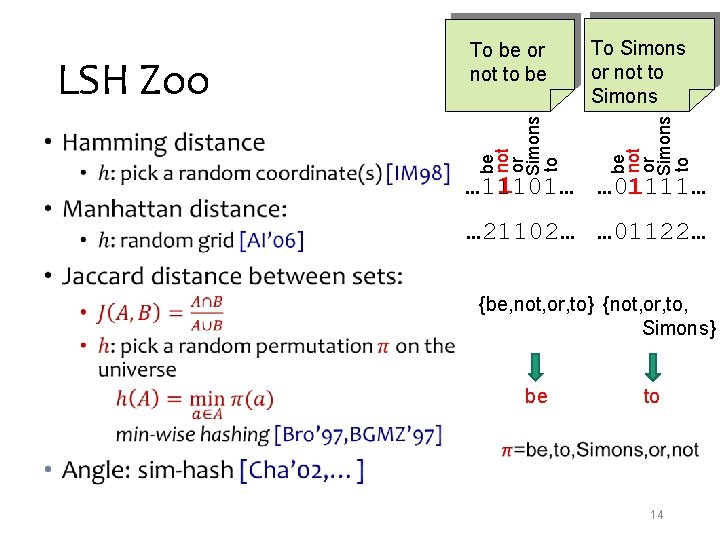To Simons or not to Simons be not or Simons to • be not or Simons to LSH Zoo To be or not to be 1 … 01111… … 11101… 1 … 21102… … 01122… {be, not, or, to} {not, or, to, Simons} be to 14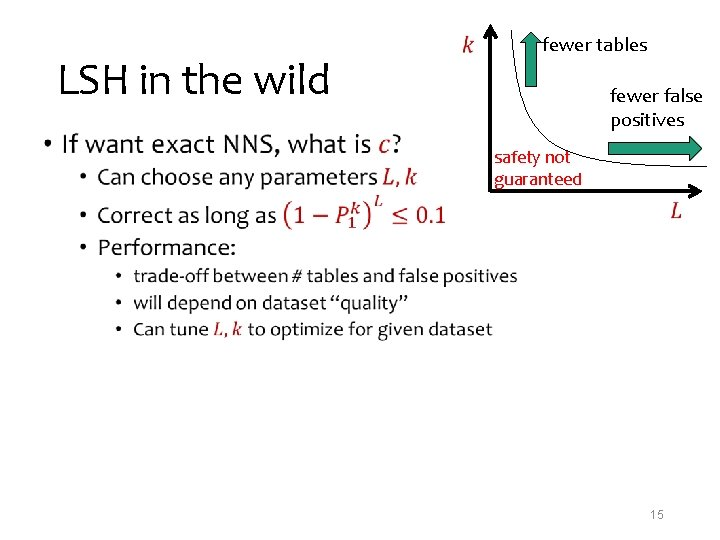LSH in the wild • fewer tables fewer false positives safety not guaranteed 15Time-Space Trade-offs space query Space time Time Comment Reference [Ind’ 01, Pan’ 06] low high [AI’ 06] [IM’ 98] [DIIM’ 04, AI’ 06] medium [MNP’ 06, OWZ’ 11] ω(1) memory lookups [PTW’ 08, PTW’ 10] p oku o l m e 1 m high low ω(1) memory lookups [KOR’ 98, IM’ 98, Pan’ 06] [AIP’ 06]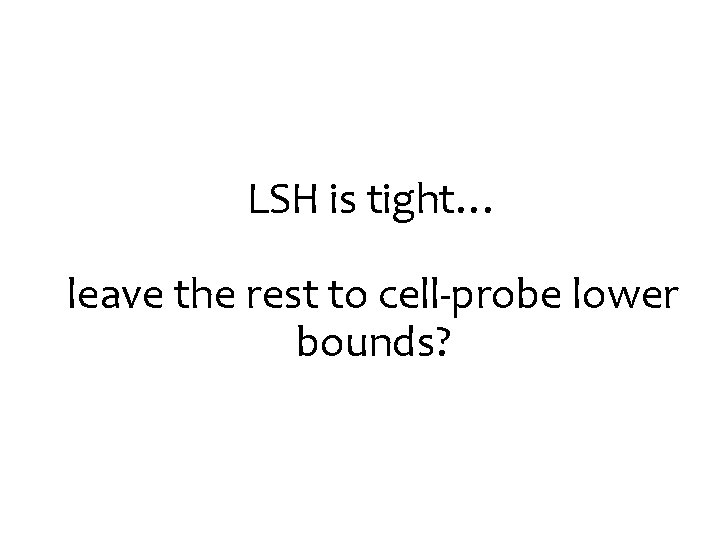LSH is tight… leave the rest to cell-probe lower bounds?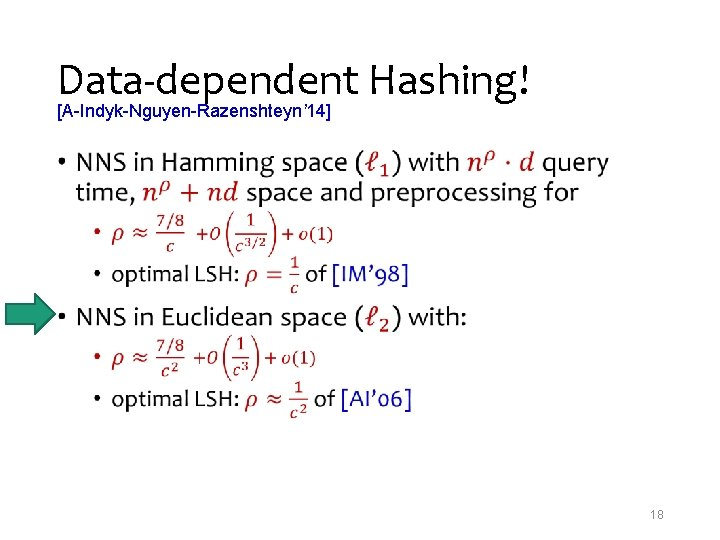Data-dependent Hashing! [A-Indyk-Nguyen-Razenshteyn’ 14] • 18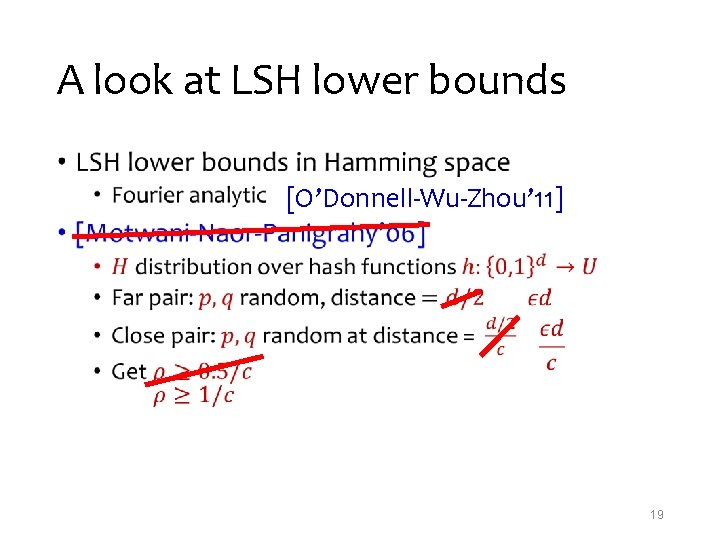A look at LSH lower bounds • [O’Donnell-Wu-Zhou’ 11] 19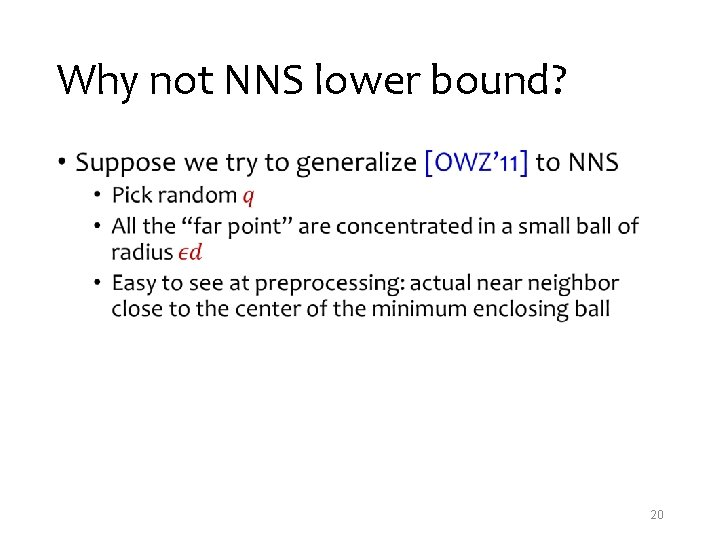Why not NNS lower bound? • 20Intuition • 21Nice Configuration: “sparsity” • 22Reduction: into spherical LSH • 23Two-level algorithm •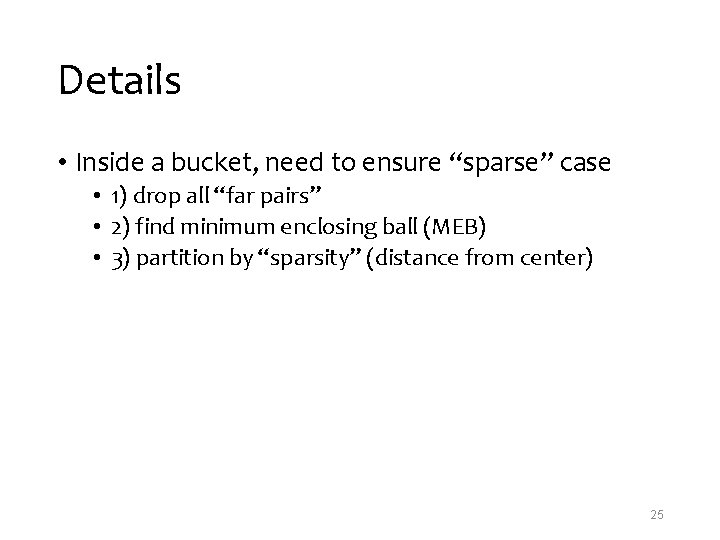Details • Inside a bucket, need to ensure “sparse” case • 1) drop all “far pairs” • 2) find minimum enclosing ball (MEB) • 3) partition by “sparsity” (distance from center) 25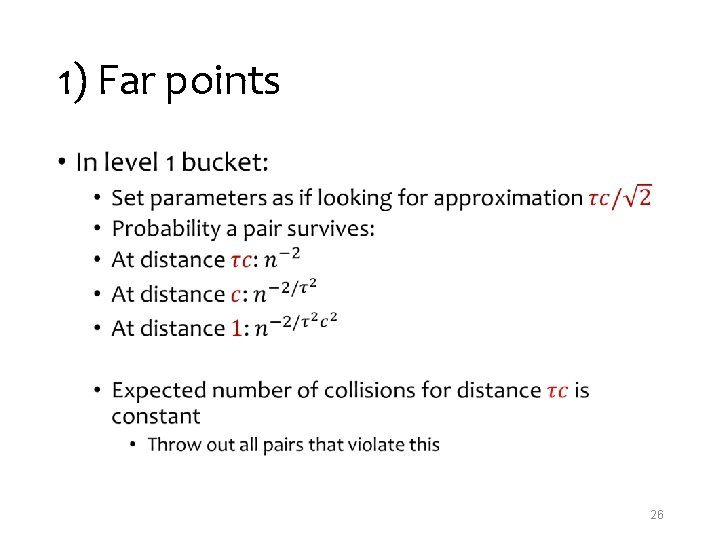1) Far points • 26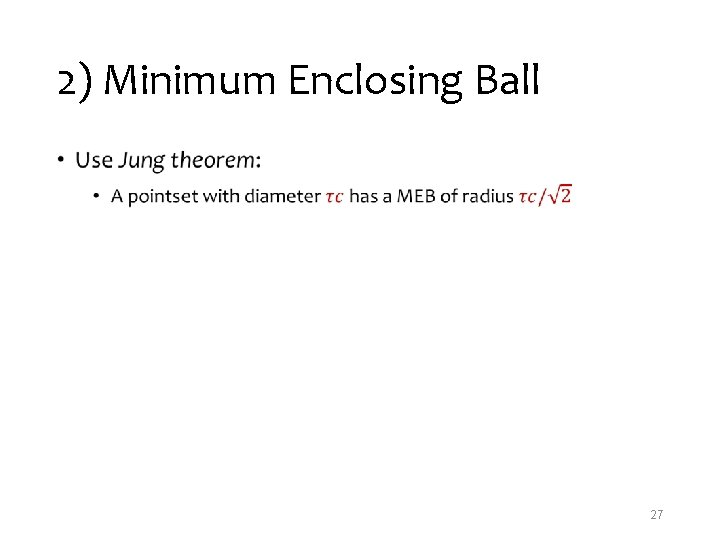2) Minimum Enclosing Ball • 27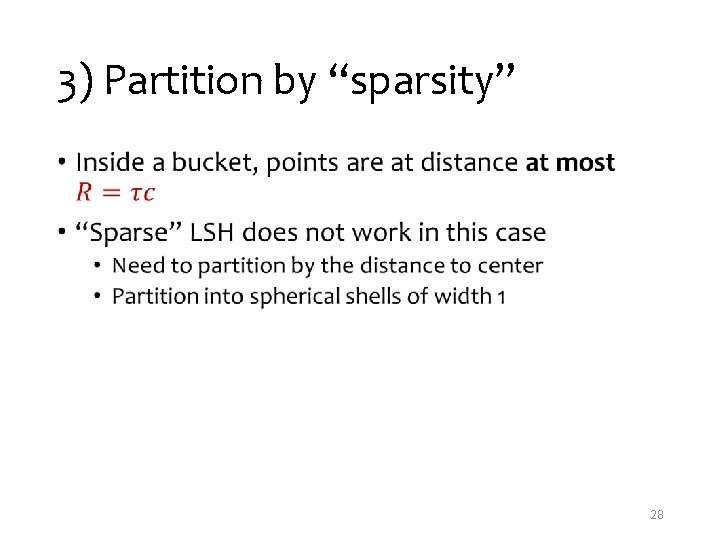3) Partition by “sparsity” • 28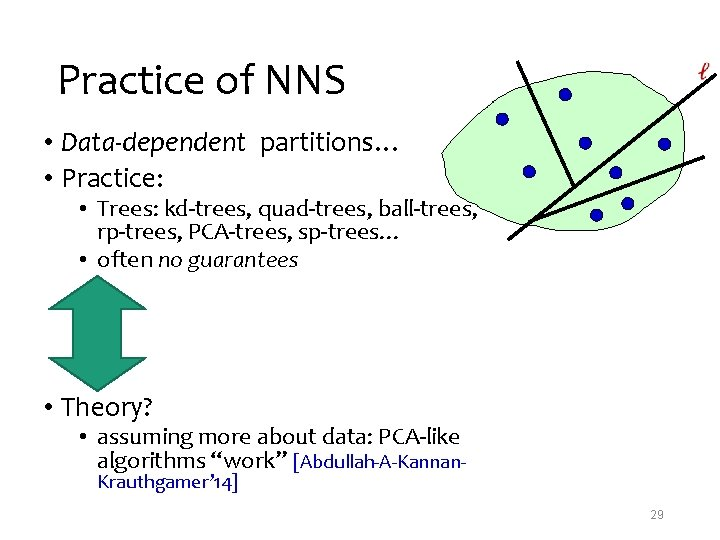Practice of NNS • Data-dependent partitions… • Practice: • Trees: kd-trees, quad-trees, ball-trees, rp-trees, PCA-trees, sp-trees… • often no guarantees • Theory? • assuming more about data: PCA-like algorithms “work” [Abdullah-A-Kannan. Krauthgamer’ 14] 29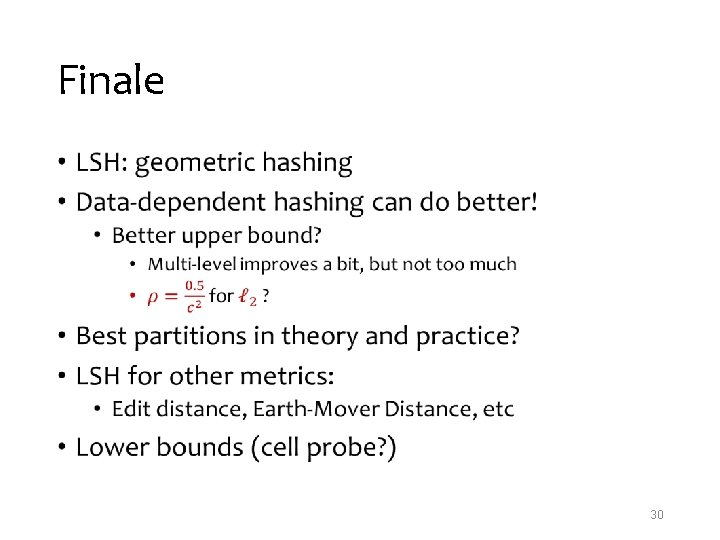Finale • 30Open question: • [Prob. needle of length 1 is not cut] ≥ 1/c 2 [Prob needle of length c is not cut]